# NumPy教程

### 概述

• NumPy是一个Python库，每个数据科学专业人员都应该熟悉它

• 这个全面的NumPy教程从头开始介绍NumPy，从基本的数学运算到NumPy如何处理图像数据

• 本文中有大量的Numpy概念和Python代码

### 介绍### 目录

• NumPy库是什么？

• Python列表与NumPy数组有什么区别？

• 创建NumPy数组

• 基本的ndarray
• 全零数组
• 全一数组
• ndarray中的随机数
• 定制的数组
• NumPy的Imatrix
• 等间距的ndarray
• NumPy数组的形状与重塑

• NumPy数组的维数
• NumPy数组的形状
• NumPy数组的大小
• 重塑NumPy数组
• 展开NumPy数组
• NumPy数组的转置
• 扩展和压缩一个NumPy数组

• 展开NumPy数组
• 压缩NumPy数组
• NumPy数组的索引与切片

• 一维数组的切片
• 二维数组切片
• 三维数组切片
• NumPy数组的负切片
• 堆叠和级联Numpy数组

• 堆叠ndarrays
• 级联ndarrays
• Numpy数组广播

• NumPy Ufuncs

• 用NumPy数组计算

• 平均值、中位数和标准差
• 最小最大值及其索引
• 在NumPy数组中排序

• NumPy数组和图像

### NumPy库是什么？

NumPy是Python数值库，是Python编程中最有用的科学库之一。它支持大型多维数组对象和各种工具。各种其他的库，如Pandas、Matplotlib和Scikit-learn，都建立在这个令人惊叹的库之上。

NumPy数组称为ndarray或N维数组，它们存储相同类型和大小的元素。它以其高性能而闻名，并在数组规模不断扩大时提供高效的存储和数据操作。

pip install numpy

import numpy as np

np实际上是数据科学界使用的NumPy的缩写。

### Python列表与NumPy数组有什么区别？

Python对象实际上是一个指向内存位置的指针，该内存位置存储有关该对象的所有详细信息，如字节和值。尽管这些额外的信息使Python成为一种动态类型语言，但它也付出了代价，这在存储大量对象（如在数组中）时变得显而易见。

Python列表本质上是一个指针数组，每个指针指向一个包含与元素相关信息的位置。这在内存和计算方面增加了很多开销。当列表中存储的所有对象都是同一类型时，大多数信息都是冗余的！

### 创建NumPy数组

#### 基本的ndarray

np.array([1,2,3,4])

array([1, 2, 3, 4])

np.array([1,2,3,4],dtype=np.float32)

array([1., 2., 3., 4.], dtype=float32)

np.array([1,2.0,3,4])

array([1., 2., 3., 4.])

NumPy数组也可以是多维的。

np.array([[1,2,3,4],[5,6,7,8]])
array([[1, 2, 3, 4],
[5, 6, 7, 8]])

#### 全零数组

NumPy允许你使用 np.zeros()方法。你只需传递所需数组的形状：

np.zeros(5)
array([0., 0., 0., 0., 0.])

np.zeros((2,3))
array([[0., 0., 0.],
[0., 0., 0.]])

#### 全一数组

np.ones(5,dtype=np.int32)
array([1, 1, 1, 1, 1])

#### ndarray中的随机数

# 随机的
np.random.rand(2,3)
array([[0.95580785, 0.98378873, 0.65133872],
[0.38330437, 0.16033608, 0.13826526]])

#### 定制的数组

np.full((2,2),7)
array([[7, 7],
[7, 7]])

#### NumPy的Imatrix

# 单位矩阵
np.eye(3)
array([[1., 0., 0.],
[0., 1., 0.],
[0., 0., 1.]])

# 不是单位矩阵
np.eye(3,k=1)
array([[0., 1., 0.],
[0., 0., 1.],
[0., 0., 0.]])

np.eye(3,k=-2)
array([[0., 0., 0.],
[0., 0., 0.],
[1., 0., 0.]])

#### 等间距的ndarray

np.arange(5)
array([0, 1, 2, 3, 4])

np.arange(2,10,2)
array([2, 4, 6, 8])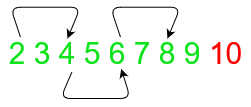np.linspace(0,1,5)
array([0.  , 0.25, 0.5 , 0.75, 1.  ])

### NumPy数组的形状与重塑

#### NumPy数组的维数

# 轴数
a = np.array([[5,10,15],[20,25,20]])
print('Array :','\n',a)
print('Dimensions :','\n',a.ndim)
Array :
[[ 5 10 15]
[20 25 20]]
Dimensions :
2

#### NumPy数组的形状

a = np.array([[1,2,3],[4,5,6]])
print('Array :','\n',a)
print('Shape :','\n',a.shape)
print('Rows = ',a.shape)
print('Columns = ',a.shape)
Array :
[[1 2 3]
[4 5 6]]
Shape :
(2, 3)
Rows =  2
Columns =  3

#### NumPy数组的大小

# size of array
a = np.array([[5,10,15],[20,25,20]])
print('Size of array :',a.size)
print('Manual determination of size of array :',a.shape*a.shape)
Size of array : 6
Manual determination of size of array : 6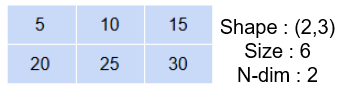#### 重塑NumPy数组

# 重塑
a = np.array([3,6,9,12])
np.reshape(a,(2,2))
array([[ 3,  6],
[ 9, 12]])

a = np.array([3,6,9,12,18,24])
print('Three rows :','\n',np.reshape(a,(3,-1)))
print('Three columns :','\n',np.reshape(a,(-1,3)))
Three rows :
[[ 3  6]
[ 9 12]
[18 24]]
Three columns :
[[ 3  6  9]
[12 18 24]]

#### 展开NumPy数组

a = np.ones((2,2))
b = a.flatten()
c = a.ravel()
print('Original shape :', a.shape)
print('Array :','\n', a)
print('Shape after flatten :',b.shape)
print('Array :','\n', b)
print('Shape after ravel :',c.shape)
print('Array :','\n', c)
Original shape : (2, 2)
Array :
[[1. 1.]
[1. 1.]]
Shape after flatten : (4,)
Array :
[1. 1. 1. 1.]
Shape after ravel : (4,)
Array :
[1. 1. 1. 1.]

b = 0
print(a)
[[1. 1.]
[1. 1.]]

c = 0
print(a)
[[0. 1.]
[1. 1.]]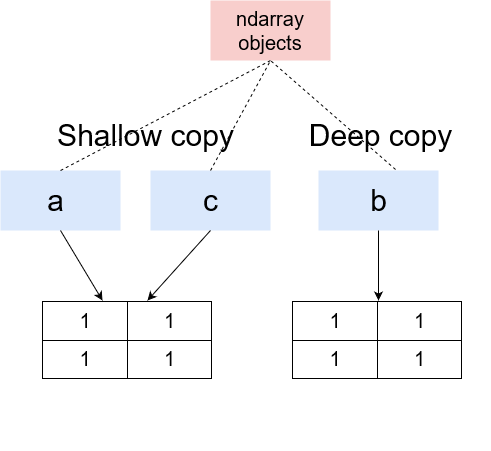#### NumPy数组的转置

NumPy的另一个非常有趣的重塑方法是transpose()方法。它接受输入数组并用列值交换行，用行值交换列值：

a = np.array([[1,2,3],
[4,5,6]])
b = np.transpose(a)
print('Original','\n','Shape',a.shape,'\n',a)
print('Expand along columns:','\n','Shape',b.shape,'\n',b)
Original
Shape (2, 3)
[[1 2 3]
[4 5 6]]
Expand along columns:
Shape (3, 2)
[[1 4]
[2 5]
[3 6]]

### 扩展和压缩一个NumPy数组

#### 展开NumPy数组

# 展开维度
a = np.array([1,2,3])
b = np.expand_dims(a,axis=0)
c = np.expand_dims(a,axis=1)
print('Original:','\n','Shape',a.shape,'\n',a)
print('Expand along columns:','\n','Shape',b.shape,'\n',b)
print('Expand along rows:','\n','Shape',c.shape,'\n',c)
Original:
Shape (3,)
[1 2 3]
Expand along columns:
Shape (1, 3)
[[1 2 3]]
Expand along rows:
Shape (3, 1)
[

]

#### 压缩NumPy数组

# squeeze
a = np.array([[[1,2,3],
[4,5,6]]])
b = np.squeeze(a, axis=0)
print('Original','\n','Shape',a.shape,'\n',a)
print('Squeeze array:','\n','Shape',b.shape,'\n',b)
Original
Shape (1, 2, 3)
[[[1 2 3]
[4 5 6]]]
Squeeze array:
Shape (2, 3)
[[1 2 3]
[4 5 6]]

# squeeze
a = np.array([[1,2,3],
[4,5,6]])
b = np.squeeze(a, axis=0)
print('Original','\n','Shape',a.shape,'\n',a)
print('Squeeze array:','\n','Shape',b.shape,'\n',b)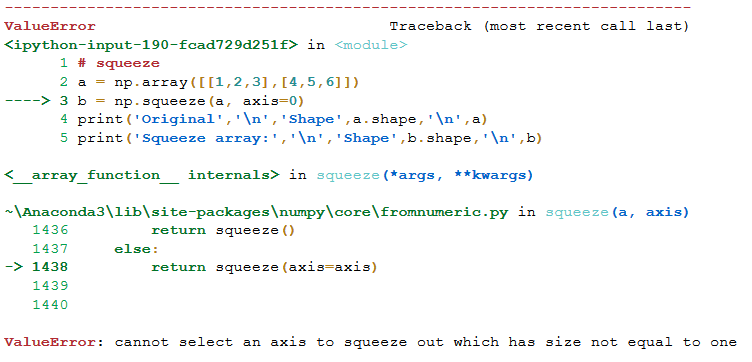### NumPy数组的索引与切片

#### 一维数组的切片

a = np.array([1,2,3,4,5,6])
print(a[1:5:2])
[2 4]

a = np.array([1,2,3,4,5,6])
print(a[1:6:2])
[2 4 6]

a = np.array([1,2,3,4,5,6])
print(a[:6:2])
print(a[1::2])
print(a[1:6:])
[1 3 5]
[2 4 6]
[2 3 4 5 6]

#### 二维数组切片

a = np.array([[1,2,3],
[4,5,6]])
print(a[0,0])
print(a[1,2])
print(a[1,0])
1
6
4

a = np.array([[1,2,3],[4,5,6]])
# 打印第一行值
print('First row values :','\n',a[0:1,:])
# 具有列的步长
print('Alternate values from first row:','\n',a[0:1,::2])
#
print('Second column values :','\n',a[:,1::2])
print('Arbitrary values :','\n',a[0:1,1:3])
First row values :
[[1 2 3]]
Alternate values from first row:
[[1 3]]
Second column values :
[
]
Arbitrary values :
[[2 3]]

#### 三维数组切片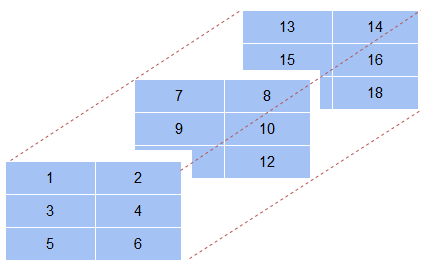a = np.array([[[1,2],[3,4],[5,6]],# 第一个轴数组
[[7,8],[9,10],[11,12]],# 第二个轴数组
[[13,14],[15,16],[17,18]]])# 第三个轴数组
# 3-D array
print(a)
[[[ 1  2]
[ 3  4]
[ 5  6]]

[[ 7  8]
[ 9 10]
[11 12]]

[[13 14]
[15 16]
[17 18]]]

# value
print('First array, first row, first column value :','\n',a[0,0,0])
print('First array last column :','\n',a[0,:,1])
print('First two rows for second and third arrays :','\n',a[1:,0:2,0:2])
First array, first row, first column value :
1
First array last column :
[2 4 6]
First two rows for second and third arrays :
[[[ 7  8]
[ 9 10]]

[[13 14]
[15 16]]]

print('Printing as a single array :','\n',a[1:,0:2,0:2].flatten())
Printing as a single array :
[ 7  8  9 10 13 14 15 16]

#### NumPy数组的负切片

a = np.array([[1,2,3,4,5],
[6,7,8,9,10]])
print(a[:,-1])
[ 5 10]

print(a[:,-1:-3:-1])
[[ 5  4]
[10  9]]

a = np.array([[1,2,3,4,5],
[6,7,8,9,10]])
print('Original array :','\n',a)
print('Reversed array :','\n',a[::-1,::-1])
Original array :
[[ 1  2  3  4  5]
[ 6  7  8  9 10]]
Reversed array :
[[10  9  8  7  6]
[ 5  4  3  2  1]]

a = np.array([[1,2,3,4,5],
[6,7,8,9,10]])
print('Original array :','\n',a)
print('Reversed array vertically :','\n',np.flip(a,axis=1))
print('Reversed array horizontally :','\n',np.flip(a,axis=0))
Original array :
[[ 1  2  3  4  5]
[ 6  7  8  9 10]]
Reversed array vertically :
[[ 5  4  3  2  1]
[10  9  8  7  6]]
Reversed array horizontally :
[[ 6  7  8  9 10]
[ 1  2  3  4  5]]

### 堆叠和级联Numpy数组

#### 堆叠ndarrays

• 使用vstack()方法垂直组合数组（即沿行），从而增加结果数组中的行数

• 或者使用hstack()以水平方式（即沿列）组合数组，从而增加结果数组中的列数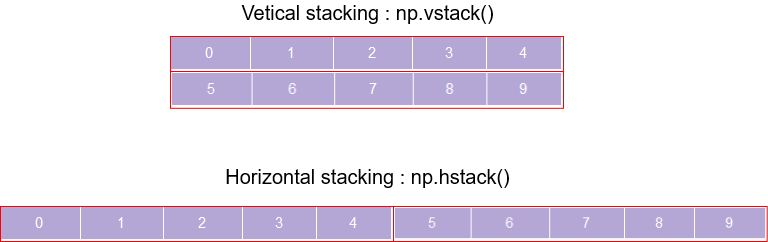a = np.arange(0,5)
b = np.arange(5,10)
print('Array 1 :','\n',a)
print('Array 2 :','\n',b)
print('Vertical stacking :','\n',np.vstack((a,b)))
print('Horizontal stacking :','\n',np.hstack((a,b)))
Array 1 :
[0 1 2 3 4]
Array 2 :
[5 6 7 8 9]
Vertical stacking :
[[0 1 2 3 4]
[5 6 7 8 9]]
Horizontal stacking :
[0 1 2 3 4 5 6 7 8 9]

a = np.arange(0,5)
b = np.arange(5,9)
print('Array 1 :','\n',a)
print('Array 2 :','\n',b)
print('Vertical stacking :','\n',np.vstack((a,b)))
print('Horizontal stacking :','\n',np.hstack((a,b)))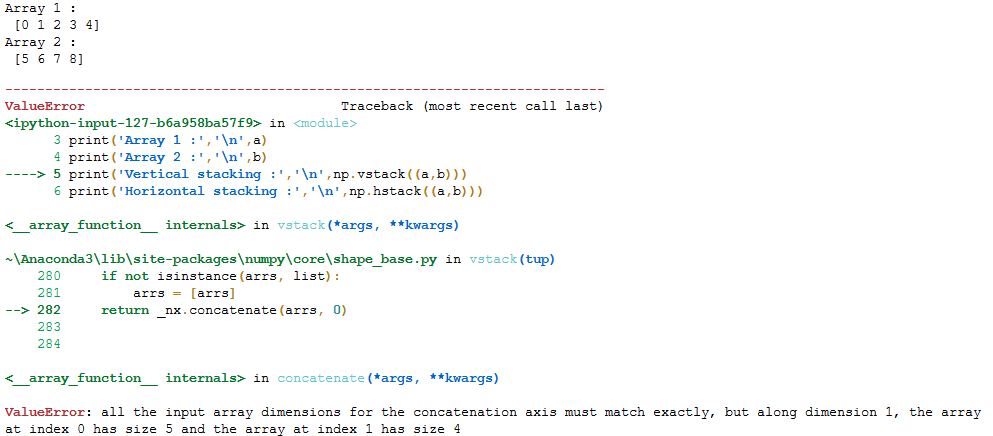a = [[1,2],[3,4]]
b = [[5,6],[7,8]]
c = np.dstack((a,b))
print('Array 1 :','\n',a)
print('Array 2 :','\n',b)
print('Dstack :','\n',c)
print(c.shape)
Array 1 :
[[1, 2], [3, 4]]
Array 2 :
[[5, 6], [7, 8]]
Dstack :
[[[1 5]
[2 6]]

[[3 7]
[4 8]]]
(2, 2, 2)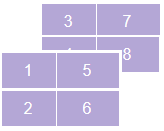#### 级联ndarrays

a = np.arange(0,5).reshape(1,5)
b = np.arange(5,10).reshape(1,5)
print('Array 1 :','\n',a)
print('Array 2 :','\n',b)
print('Concatenate along rows :','\n',np.concatenate((a,b),axis=0))
print('Concatenate along columns :','\n',np.concatenate((a,b),axis=1))
Array 1 :
[[0 1 2 3 4]]
Array 2 :
[[5 6 7 8 9]]
Concatenate along rows :
[[0 1 2 3 4]
[5 6 7 8 9]]
Concatenate along columns :
[[0 1 2 3 4 5 6 7 8 9]]

# 将值附加到ndarray
a = np.array([[1,2],
[3,4]])
np.append(a,[[5,6]], axis=0)
array([[1, 2],
[3, 4],
[5, 6]])

### Numpy数组广播

a = np.arange(10,20,2)
b = np.array([,])
print('Adding two different size arrays :','\n',a+b)
print('Multiplying an ndarray and a number :',a*2)
Adding two different size arrays :
[[12 14 16 18 20]
[12 14 16 18 20]]
Multiplying an ndarray and a number : [20 24 28 32 36]

Ndarrays在以下情况下兼容：

1. 两者都有相同的尺寸

2. 其中一个ndarrays的维数是1。尺寸为1的广播会满足尺寸更大的ndarray的大小要求

a = np.ones((3,3))
b = np.array()
a+b
array([[3., 3., 3.],
[3., 3., 3.],
[3., 3., 3.]])

### NumPy Ufuncs

Python是一种动态类型语言。这意味着在赋值时不需要知道变量的数据类型。Python将在运行时自动确定它。虽然这意味着编写更干净、更容易的代码，但也会使Python变得迟缓。

NumPy使这个工作更快的方法是使用向量化。向量化在编译的代码中以逐元素的方式对ndarray执行相同的操作。因此，不需要每次都确定元素的数据类型，从而执行更快的操作。

ufuncs 是NumPy中的通用函数，只是数学函数。它们执行快速的元素功能。当对NumPy数组执行简单的算术操作时，它们会自动调用，因为它们充当NumPy ufuncs的包装器。

a = [1,2,3,4,5]
b = [6,7,8,9,10]
%timeit a+b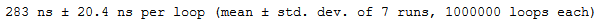a = np.arange(1,6)
b = np.arange(6,11)
%timeit a+b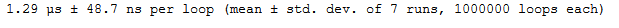### 用NumPy数组计算

#### NumPy数组的基本运算

print('Subtract :',a-5)
print('Multiply :',a*5)
print('Divide :',a/5)
print('Power :',a**2)
print('Remainder :',a%5)
Subtract : [-4 -3 -2 -1 0]
Multiply : [ 5 10 15 20 25]
Divide : [0.2 0.4 0.6 0.8 1. ]
Power : [ 1 4 9 16 25]
Remainder : [1 2 3 4 0]

#### 平均值、中位数和标准差

a = np.arange(5,15,2)
print('Mean :',np.mean(a))
print('Standard deviation :',np.std(a))
print('Median :',np.median(a))
Mean : 9.0
Standard deviation : 2.8284271247461903
Median : 9.0

#### 最小最大值及其索引

a = np.array([[1,6],
[4,3]])
# 最小值
print('Min :',np.min(a,axis=0))
# 最大值
print('Max :',np.max(a,axis=1))
Min : [1 3]
Max : [6 4]

a = np.array([[1,6,5],
[4,3,7]])
# 最小值
print('Min :',np.argmin(a,axis=0))
# 最大值
print('Max :',np.argmax(a,axis=1))
Min : [0 1 0]
Max : [1 2]

### 在NumPy数组中排序

a = np.array([1,4,2,5,3,6,8,7,9])
np.sort(a, kind='quicksort')
array([1, 2, 3, 4, 5, 6, 7, 8, 9])

a = np.array([[5,6,7,4],
[9,2,3,7]])# 沿列排序
print('Sort along column :','\n',np.sort(a, kind='mergresort',axis=1))
# 沿行排序
print('Sort along row :','\n',np.sort(a, kind='mergresort',axis=0))
Sort along column :
[[4 5 6 7]
[2 3 7 9]]
Sort along row :
[[5 2 3 4]
[9 6 7 7]]

### NumPy数组和图像

NumPy数组在存储和操作图像数据方面有着广泛的用途。但图像数据到底是什么呢？import numpy as np
import matplotlib.pyplot as plt
from scipy import misc

# 读取图像
# 图像
im
array([[[115, 106,  67],
[113, 104,  65],
[112, 103,  64],
...,
[160, 138,  37],
[160, 138,  37],
[160, 138,  37]],

[[117, 108,  69],
[115, 106,  67],
[114, 105,  66],
...,
[157, 135,  36],
[157, 135,  34],
[158, 136,  37]],

[[120, 110,  74],
[118, 108,  72],
[117, 107,  71],
...,

print(im.shape)
print(type(type))
(561, 997, 3)
numpy.ndarray

# 翻转
plt.imshow(np.flip(im, axis=1))im/255
array([[[0.45098039, 0.41568627, 0.2627451 ],
[0.44313725, 0.40784314, 0.25490196],
[0.43921569, 0.40392157, 0.25098039],
...,
[0.62745098, 0.54117647, 0.14509804],
[0.62745098, 0.54117647, 0.14509804],
[0.62745098, 0.54117647, 0.14509804]],

[[0.45882353, 0.42352941, 0.27058824],
[0.45098039, 0.41568627, 0.2627451 ],
[0.44705882, 0.41176471, 0.25882353],
...,
[0.61568627, 0.52941176, 0.14117647],
[0.61568627, 0.52941176, 0.13333333],
[0.61960784, 0.53333333, 0.14509804]],

[[0.47058824, 0.43137255, 0.29019608],
[0.4627451 , 0.42352941, 0.28235294],
[0.45882353, 0.41960784, 0.27843137],
...,
[0.6       , 0.52156863, 0.14117647],
[0.6       , 0.52156863, 0.13333333],
[0.6       , 0.52156863, 0.14117647]],

...,